# Multiplicity of positive solutions for eigenvalue problems of $\left(p,2\right)$-equations

## Abstract

We consider a nonlinear parametric equation driven by the sum of a p-Laplacian ($p>2$) and a Laplacian (a $\left(p,2\right)$-equation) with a Carathéodory reaction, which is strictly $\left(p-2\right)$-sublinear near +∞. Using variational methods coupled with truncation and comparison techniques, we prove a bifurcation-type theorem for the nonlinear eigenvalue problem. So, we show that there is a critical parameter value ${\lambda }_{\ast }>0$ such that for $\lambda >{\lambda }_{\ast }$ the problem has at least two positive solutions, if $\lambda ={\lambda }_{\ast }$, then the problem has at least one positive solution and for $\lambda \in \left(0,{\lambda }_{\ast }\right)$, it has no positive solutions.

MSC: 35J25, 35J92.

## 1 Introduction

Let $\mathrm{\Omega }\subseteq {\mathbb{R}}^{N}$ be a bounded domain with a ${C}^{2}$-boundary Ω. In this paper, we study the following nonlinear Dirichlet eigenvalue problem: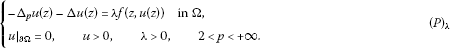Here, by ${\mathrm{\Delta }}_{p}$ we denote the p-Laplace differential operator defined by

${\mathrm{\Delta }}_{p}u\left(z\right)=div\left({\parallel \mathrm{\nabla }u\left(z\right)\parallel }^{p-2}\mathrm{\nabla }u\left(z\right)\right)\phantom{\rule{1em}{0ex}}\mathrm{\forall }u\in {W}_{0}^{1,p}\left(\mathrm{\Omega }\right)$

(with $2). In ${\left(P\right)}_{\lambda }$, $\lambda >0$ is a parameter and $f\left(z,\zeta \right)$ is a Carathéodory function (i.e., for all $\zeta \in \mathbb{R}$, the function $z↦f\left(z,\zeta \right)$ is measurable and for almost all $z\in \mathrm{\Omega }$, the function $\zeta ↦f\left(z,\zeta \right)$ is continuous), which exhibits strictly $\left(p-2\right)$-sublinear growth in the ζ-variable near +∞. The aim of this paper is to determine the precise dependence of the set of positive solutions on the parameter $\lambda >0$. So, we prove a bifurcation-type theorem, which establishes the existence of a critical parameter value ${\lambda }_{\ast }>0$ such that for all $\lambda >{\lambda }_{\ast }$, problem ${\left(P\right)}_{\lambda }$ has at least two nontrivial positive smooth solutions, for $\lambda ={\lambda }_{\ast }$, problem ${\left(P\right)}_{\lambda }$ has at least one nontrivial positive smooth solution and for $\lambda \in \left(0,{\lambda }_{\ast }\right)$, problem ${\left(P\right)}_{\lambda }$ has no positive solution. Similar nonlinear eigenvalue problems with $\left(p-2\right)$-sublinear reaction were studied by Maya and Shivaji  and Rabinowitz  for problems driven by the Laplacian and by Guo , Hu and Papageorgiou  and Perera  for problems driven by the p-Laplacian. However, none of the aforementioned works produces the precise dependence of the set of positive solutions on the parameter $\lambda >0$ (i.e., they do not prove a bifurcation-type theorem). We mention that in problem ${\left(P\right)}_{\lambda }$ the differential operator is not homogeneous in contrast to the case of the Laplacian and p-Laplacian. This fact is the source of difficulties in the study of problem ${\left(P\right)}_{\lambda }$ which lead to new tools and methods.

We point out that $\left(p,2\right)$-equations (i.e., equations in which the differential operator is the sum of a p-Laplacian and a Laplacian) are important in quantum physics in the search for solitions. We refer to the work of Benci, D’Avenia-Fortunato and Pisani . More recently, there have been some existence and multiplicity results for such problems; see Cingolani and Degiovanni , Sun . Finally, we should mention the recent papers of Marano and Papageorgiou [9, 10]. In  the authors deal with parametric p-Laplacian equations in which the reaction exhibits competing nonlinearities (concave-convex nonlinearity). In , they study a nonparametric $\left(p,q\right)$-equation with a reaction that has different behavior both at ±∞ and at 0 from those considered in the present paper, and so the geometry of the problem is different.

Out approach is variational based on the critical point theory, combined with suitable truncation and comparison techniques. In the next section, for the convenience of the reader, we briefly recall the main mathematical tools that we use in this paper.

## 2 Mathematical background

Let X be a Banach space and let ${X}^{\ast }$ be its topological dual. By $〈\cdot ,\cdot 〉$ we denote the duality brackets for the pair $\left({X}^{\ast },X\right)$. Let $\phi \in {C}^{1}\left(X\right)$. A point ${x}_{0}\in X$ is a critical point of φ if ${\phi }^{\prime }\left({x}_{0}\right)=0$. A number $c\in \mathbb{R}$ is a critical value of φ if there exists a critical point ${x}_{0}\in X$ such that $\phi \left({x}_{0}\right)=c$.

We say that $\phi \in {C}^{1}\left(X\right)$ satisfies the Palais-Smale condition if the following is true:

‘Every sequence ${\left\{{x}_{n}\right\}}_{n⩾1}\subseteq X$, such that ${\left\{\phi \left({x}_{n}\right)\right\}}_{n⩾1}\subseteq \mathbb{R}$ is bounded and

This compactness-type condition is crucial in proving a deformation theorem which in turn leads to the minimax theory of certain critical values of $\phi \in {C}^{1}\left(X\right)$ (see, e.g., Gasinski and Papageorgiou ). A well-written discussion of this compactness condition and its role in critical point theory can be found in Mawhin and Willem . One of the minimax theorems needed in the sequel is the well-known ‘mountain pass theorem’.

Theorem 2.1 If $\phi \in {C}^{1}\left(X\right)$ satisfies the Palais-Smale condition, ${x}_{0},{x}_{1}\in X$, $\parallel {x}_{1}-{x}_{0}\parallel >r>0$,

$max\left\{\phi \left({x}_{0}\right),\phi \left({x}_{1}\right)\right\}

and

$c=\underset{\gamma \in \mathrm{\Gamma }}{inf}\underset{0⩽t⩽1}{max}\phi \left(\gamma \left(t\right)\right),$

where

$\mathrm{\Gamma }=\left\{\gamma \in C\left(\left[0,1\right];X\right):\gamma \left(0\right)={x}_{0},\gamma \left(1\right)={x}_{1}\right\},$

then $c⩾{\eta }_{r}$ and c is a critical value of φ.

In the analysis of problem ${\left(P\right)}_{\lambda }$, in addition to the Sobolev space ${W}_{0}^{1,p}\left(\mathrm{\Omega }\right)$, we will also use the Banach space

${C}_{0}^{1}\left(\overline{\mathrm{\Omega }}\right)=\left\{u\in {C}^{1}\left(\overline{\mathrm{\Omega }}\right):u{|}_{\partial \mathrm{\Omega }}=0\right\}.$

This is an ordered Banach space with a positive cone:

This cone has a nonempty interior given by

where by $n\left(\cdot \right)$ we denote the outward unit normal on Ω.

Let ${f}_{0}:\mathrm{\Omega }×\mathbb{R}⟶\mathbb{R}$ be a Carathéodory function with subcritical growth in $\zeta \in \mathbb{R}$, i.e.,

with ${a}_{0}\in {L}^{\mathrm{\infty }}{\left(\mathrm{\Omega }\right)}_{+}$, ${c}_{0}>0$ and $1, where

(the critical Sobolev exponent).

We set

${F}_{0}\left(z,\zeta \right)={\int }_{0}^{\zeta }{f}_{0}\left(z,s\right)\phantom{\rule{0.2em}{0ex}}ds$

and consider the ${C}^{1}$-functional ${\psi }_{0}:{W}_{0}^{1,p}\left(\mathrm{\Omega }\right)⟶\mathbb{R}$ defined by

${\psi }_{0}\left(u\right)=\frac{1}{p}{\parallel \mathrm{\nabla }u\parallel }_{p}^{p}+\frac{1}{2}{\parallel \mathrm{\nabla }u\parallel }_{2}^{2}-{\int }_{\mathrm{\Omega }}{F}_{0}\left(z,u\left(z\right)\right)\phantom{\rule{0.2em}{0ex}}dz\phantom{\rule{1em}{0ex}}\mathrm{\forall }u\in {W}_{0}^{1,p}\left(\mathrm{\Omega }\right).$
(2.1)

The next proposition is a special case of a more general result proved by Gasinski and Papageorgiou . We mention that the first result of this type was proved by Brezis and Nirenberg .

Proposition 2.2 If ${\psi }_{0}$ is defined by (2.1) and ${u}_{0}\in {W}_{0}^{1,p}\left(\mathrm{\Omega }\right)$ is a local ${C}_{0}^{1}\left(\overline{\mathrm{\Omega }}\right)$-minimizer of ${\psi }_{0}$, i.e., there exists ${\varrho }_{1}>0$ such that

${\psi }_{0}\left({u}_{0}\right)⩽{\psi }_{0}\left({u}_{0}+h\right)\phantom{\rule{1em}{0ex}}\mathrm{\forall }h\in {C}_{0}^{1}\left(\overline{\mathrm{\Omega }}\right),{\parallel h\parallel }_{{C}_{0}^{1}\left(\overline{\mathrm{\Omega }}\right)}⩽{\varrho }_{1},$

then ${u}_{0}\in {C}_{1}^{1,\beta }\left(\overline{\mathrm{\Omega }}\right)$ for some $\beta \in \left(0,1\right)$ and ${u}_{0}$ is also a local ${W}_{0}^{1,p}\left(\mathrm{\Omega }\right)$-minimizer of ${\psi }_{0}$, i.e., there exists ${\varrho }_{2}>0$ such that

${\psi }_{0}\left({u}_{0}\right)⩽{\psi }_{0}\left({u}_{0}+h\right)\phantom{\rule{1em}{0ex}}\mathrm{\forall }h\in {W}_{0}^{1,p}\left(\mathrm{\Omega }\right),\parallel h\parallel ⩽{\varrho }_{2}.$

Let $g,h\in {L}^{\mathrm{\infty }}\left(\mathrm{\Omega }\right)$. We say that $g\prec h$ if for all compact subsets $K\subseteq \mathrm{\Omega }$, we can find $\epsilon =\epsilon \left(K\right)>0$ such that

Clearly, if $g,h\in C\left(\mathrm{\Omega }\right)$ and $g\left(z\right) for all $z\in \mathrm{\Omega }$, then $g\prec h$. A slight modification of the proof of Proposition 2.6 of Arcoya and Ruiz  in order to accommodate the presence of the extra linear term $-\mathrm{\Delta }u$ leads to the following strong comparison principle.

Proposition 2.3 If $\xi ⩾0$, $g,h\in {L}^{\mathrm{\infty }}\left(\mathrm{\Omega }\right)$, $g\prec h$ and $u\in {C}_{0}^{1}\left(\overline{\mathrm{\Omega }}\right)$, $v\in int{C}_{+}$ are solutions of the problems

$\left\{\begin{array}{c}-{\mathrm{\Delta }}_{p}u\left(z\right)-\mathrm{\Delta }u\left(z\right)+\xi {|u\left(z\right)|}^{p-2}u\left(z\right)=g\left(z\right)\phantom{\rule{1em}{0ex}}\mathit{\text{in}}\mathrm{\Omega },\hfill \\ -{\mathrm{\Delta }}_{p}v\left(z\right)-\mathrm{\Delta }v\left(z\right)+\xi {|v\left(z\right)|}^{p-2}v\left(z\right)=h\left(z\right)\phantom{\rule{1em}{0ex}}\mathit{\text{in}}\mathrm{\Omega },\hfill \end{array}$

then $v-u\in int{C}_{+}$.

Let $r\in \left(1,+\mathrm{\infty }\right)$ and let ${A}_{r}:{W}_{0}^{1,r}\left(\mathrm{\Omega }\right)⟶{W}^{-1,{r}^{\prime }}\left(\mathrm{\Omega }\right)={W}_{0}^{1,r}{\left(\mathrm{\Omega }\right)}^{\ast }$ (where $\frac{1}{r}+\frac{1}{{r}^{\prime }}=1$) be a nonlinear map defined by

$〈{A}_{r}\left(u\right),y〉={\int }_{\mathrm{\Omega }}{\parallel \mathrm{\nabla }u\parallel }^{r-2}{\left(\mathrm{\nabla }u,\mathrm{\nabla }y\right)}_{{\mathbb{R}}^{N}}\phantom{\rule{0.2em}{0ex}}dz\phantom{\rule{1em}{0ex}}\mathrm{\forall }u,y\in {W}_{0}^{1,r}\left(\mathrm{\Omega }\right).$
(2.2)

The next proposition can be found in Dinca, Jebelean and Mawhin  and Gasiński and Papageorgiou .

Proposition 2.4 If ${A}_{r}:{W}_{0}^{1,r}\left(\mathrm{\Omega }\right)⟶{W}^{-1,{r}^{\prime }}\left(\mathrm{\Omega }\right)$ (where $1) is defined by (2.2), then ${A}_{r}$ is continuous, strictly monotone (hence maximal monotone too), bounded and of type ${\left(S\right)}_{+}$, i.e., if ${u}_{n}⟶u$ weakly in ${W}_{0}^{1,r}\left(\mathrm{\Omega }\right)$ and

$\underset{n\to +\mathrm{\infty }}{lim sup}〈{A}_{r}\left({u}_{n}\right),{u}_{n}-u〉⩽0,$

then ${u}_{n}⟶u$ in ${W}_{0}^{1,p}\left(\mathrm{\Omega }\right)$.

If $r=2$, then we write ${A}_{2}=A\in \mathcal{L}\left({H}_{0}^{1}\left(\mathrm{\Omega }\right);{H}^{-1}\left(\mathrm{\Omega }\right)\right)$.

In what follows, by ${\stackrel{ˆ}{\lambda }}_{1}\left(p\right)$ we denote the first eigenvalue of the negative Dirichlet p-Laplacian $\left(-{\mathrm{\Delta }}_{p},{W}_{0}^{1,p}\left(\mathrm{\Omega }\right)\right)$. We know that ${\stackrel{ˆ}{\lambda }}_{1}\left(p\right)>0$ and it admits the following variational characterization:

${\stackrel{ˆ}{\lambda }}_{1}\left(p\right)=inf\left\{\frac{{\parallel \mathrm{\nabla }u\parallel }_{p}^{p}}{{\parallel u\parallel }_{p}^{p}}:u\in {W}_{0}^{1,p}\left(\mathrm{\Omega }\right),u\ne 0\right\}.$
(2.3)

Finally, throughout this work, by $\parallel \cdot \parallel$ we denote the norm of the Sobolev space ${W}_{0}^{1,p}\left(\mathrm{\Omega }\right)$. By virtue of the Poincaré inequality, we have

$\parallel u\parallel ={\parallel \mathrm{\nabla }u\parallel }_{p}\phantom{\rule{1em}{0ex}}\mathrm{\forall }u\in {W}_{0}^{1,p}\left(\mathrm{\Omega }\right).$

The notation $\parallel \cdot \parallel$ will also be used to denote the norm of ${\mathbb{R}}^{N}$. No confusion is possible since it will always be clear from the context which norm is used. For $\zeta \in \mathbb{R}$, we set ${\zeta }^{±}=max\left\{±\zeta ,0\right\}$. Then for $u\in {W}_{0}^{1,p}\left(\mathrm{\Omega }\right)$, we define ${u}^{±}\left(\cdot \right)=u{\left(\cdot \right)}^{±}$. We know that

${u}^{±}\in {W}_{0}^{1,p}\left(\mathrm{\Omega }\right),\phantom{\rule{1em}{0ex}}|u|={u}^{+}+{u}^{-},\phantom{\rule{2em}{0ex}}u={u}^{+}-{u}^{-}\phantom{\rule{1em}{0ex}}\mathrm{\forall }u\in {W}_{0}^{1,p}\left(\mathrm{\Omega }\right).$

If $h:\mathrm{\Omega }×\mathbb{R}⟶\mathbb{R}$ is superpositionally measurable (for example, a Carathéodory function), then we set

${N}_{h}\left(u\right)\left(\cdot \right)=h\left(\cdot ,u\left(\cdot \right)\right)\phantom{\rule{1em}{0ex}}\mathrm{\forall }u\in {W}_{0}^{1,p}\left(\mathrm{\Omega }\right).$

By ${|\cdot |}_{N}$ we denote the Lebesgue measure on ${\mathbb{R}}^{N}$.

## 3 Positive solutions

The hypotheses on the reaction f are the following.

H: $f:\mathrm{\Omega }×\mathbb{R}⟶\mathbb{R}$ is a Carathéodory function such that $f\left(z,0\right)=0$ for almost all $z\in \mathrm{\Omega }$, $f\left(z,\zeta \right)⩾0$ for almost all $z\in \mathrm{\Omega }$ and all $\zeta ⩾0$ and

(i) for every $\varrho >0$, there exists ${a}_{\varrho }\in {L}^{\mathrm{\infty }}{\left(\mathrm{\Omega }\right)}_{+}$ such that

(ii) ${lim}_{\zeta \to +\mathrm{\infty }}\frac{f\left(z,\zeta \right)}{{\zeta }^{p-1}}=0$ uniformly for almost all $z\in \mathrm{\Omega }$;

(iii) ${lim}_{\zeta \to {0}^{+}}\frac{f\left(z,\zeta \right)}{{\zeta }^{p-1}}=0$ uniformly for almost all $z\in \mathrm{\Omega }$;

(iv) for every $\varrho >0$, there exists ${\xi }_{\varrho }>0$ such that for almost all $z\in \mathrm{\Omega }$, the map $\zeta ↦f\left(z,\zeta \right)+{\xi }_{p}{\zeta }^{p-1}$ is nondecreasing on $\left[0,\varrho \right]$;

(v) if

$F\left(z,\zeta \right)={\int }_{0}^{\zeta }f\left(z,s\right)\phantom{\rule{0.2em}{0ex}}ds,$

then there exists $c\in \mathbb{R}$ such that

Remark 3.1 Since we are looking for positive solutions and hypotheses H concern only the positive semiaxis ${\mathbb{R}}_{+}=\left[0,+\mathrm{\infty }\right)$, we may and will assume that $f\left(z,\zeta \right)=0$ for almost all $z\in \mathrm{\Omega }$ and all $\zeta ⩽0$. Hypothesis H(ii) implies that for almost all $z\in \mathrm{\Omega }$, the map $f\left(z,\cdot \right)$ is strictly $\left(p-2\right)$-sublinear near +∞. Hypothesis H(iv) is much weaker than assuming the monotonicity of $f\left(z,\cdot \right)$ for almost all $z\in \mathrm{\Omega }$.

Example 3.2 The following functions satisfy hypotheses H (for the sake of simplicity, we drop the z-dependence):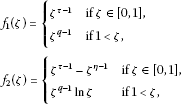with $1. Clearly ${f}_{2}$ is not monotone.

Let

and let $S\left(\lambda \right)$ be the set of solutions of ${\left(P\right)}_{\lambda }$. We set

${\lambda }_{\ast }=inf\mathcal{Y}$

(if $\mathcal{Y}=\mathrm{\varnothing }$, then ${\lambda }_{\ast }=+\mathrm{\infty }$).

Proposition 3.3 If hypotheses H hold, then

$S\left(\lambda \right)\subseteq int{C}_{+}\phantom{\rule{1em}{0ex}}\mathit{\text{and}}\phantom{\rule{1em}{0ex}}{\lambda }_{\ast }>0.$

Proof Clearly, the result is true if $\mathcal{Y}=\mathrm{\varnothing }$. So, suppose that $\mathcal{Y}\ne \mathrm{\varnothing }$ and let $\lambda \in \mathcal{Y}$. So, we can find $u\in S\left(\lambda \right)\cap {W}_{0}^{1,p}\left(\mathrm{\Omega }\right)$ such that

From Ladyzhenskaya and Uraltseva [, p.286], we have that $u\in {L}^{\mathrm{\infty }}\left(\mathrm{\Omega }\right)$. Then we can apply Theorem 1 of Lieberman  and have that $u\in int{C}_{+}\setminus \left\{0\right\}$. Let $\varrho ={\parallel u\parallel }_{\mathrm{\infty }}$ and let ${\xi }_{\varrho }>0$ be as postulated by hypothesis H(iv). Then

so

From the strong maximum principle of Pucci and Serrin [, p.34], we have that

$u\left(z\right)>0\phantom{\rule{1em}{0ex}}\mathrm{\forall }z\in \mathrm{\Omega }.$

So, we can apply the boundary point theorem of Pucci and Serrin [, p.120] and have that $u\in int{C}_{+}$. Therefore, $S\left(\lambda \right)\subseteq int{C}_{+}$.

By virtue of hypotheses H(ii) and (iii), we see that we can find ${c}_{1}>0$ such that

(3.1)

Let ${\lambda }_{0}\in \left(0,\frac{{\stackrel{ˆ}{\lambda }}_{1}\left(p\right)}{{c}_{1}}\right)$ and $\vartheta \in \left(0,{\lambda }_{0}\right]$. Suppose that $\vartheta \in \mathcal{Y}$. Then from the first part of the proof, we know that we can find ${u}_{\vartheta }\in S\left(\vartheta \right)\subseteq int{C}_{+}$. We have

${A}_{p}\left({u}_{\vartheta }\right)+A\left({u}_{\vartheta }\right)=\vartheta {N}_{f}\left({u}_{\vartheta }\right),$

so

${\parallel \mathrm{\nabla }{u}_{\vartheta }\parallel }_{p}^{p}⩽{\int }_{\mathrm{\Omega }}\vartheta f\left(z,{u}_{\vartheta }\right){u}_{\vartheta }\phantom{\rule{0.2em}{0ex}}dz⩽\vartheta {c}_{1}{\parallel {u}_{\vartheta }\parallel }_{p}^{p}⩽{\lambda }_{0}{c}_{1}{\parallel {u}_{\vartheta }\parallel }_{p}^{p}<{\stackrel{ˆ}{\lambda }}_{1}\left(p\right){\parallel {u}_{\vartheta }\parallel }_{p}^{p}$

(see (3.1) and recall that $\vartheta ⩽{\lambda }_{0}<\frac{{\stackrel{ˆ}{\lambda }}_{1}\left(p\right)}{{c}_{1}}$), which contradicts (2.3). Therefore, ${\lambda }_{\ast }⩾{\lambda }_{0}>0$. □

For $\lambda >0$, let ${\phi }_{\lambda }:{W}_{0}^{1,p}\left(\mathrm{\Omega }\right)⟶\mathbb{R}$ be the energy functional for problem ${\left(P\right)}_{\lambda }$ defined by

${\phi }_{\lambda }\left(u\right)=\frac{1}{p}{\parallel \mathrm{\nabla }u\parallel }_{p}^{p}+\frac{1}{2}{\parallel \mathrm{\nabla }u\parallel }_{2}^{2}-\lambda {\int }_{\mathrm{\Omega }}F\left(z,u\right)\phantom{\rule{0.2em}{0ex}}dz\phantom{\rule{1em}{0ex}}\mathrm{\forall }u\in {W}_{0}^{1,p}\left(\mathrm{\Omega }\right).$

Evidently, ${\phi }_{\lambda }\in C\left({W}_{0}^{1,p}\left(\mathrm{\Omega }\right)\right)$.

Proposition 3.4 If hypotheses H hold, then $\mathcal{Y}\ne \mathrm{\varnothing }$.

Proof By virtue of hypotheses H(i) and (ii), for a given $\epsilon >0$, we can find ${c}_{\epsilon }>0$ such that

(3.2)

Then for $u\in {W}_{0}^{1,p}\left(\mathrm{\Omega }\right)$ and $\lambda >0$, we have

$\begin{array}{rcl}{\phi }_{\lambda }\left(u\right)& =& \frac{1}{p}{\parallel \mathrm{\nabla }u\parallel }_{p}^{p}+\frac{1}{2}{\parallel \mathrm{\nabla }u\parallel }_{2}^{2}-\lambda {\int }_{\mathrm{\Omega }}F\left(z,u\right)\phantom{\rule{0.2em}{0ex}}dz\\ ⩾& \frac{1}{p}{\parallel \mathrm{\nabla }u\parallel }_{p}^{p}-\frac{\lambda \epsilon }{p}{\parallel {u}^{+}\parallel }_{p}^{p}-\lambda {c}_{\epsilon }{|\mathrm{\Omega }|}_{N}\\ =& \frac{1}{p}\left(1-\frac{\lambda \epsilon }{{\stackrel{ˆ}{\lambda }}_{1}\left(p\right)}\right){\parallel u\parallel }^{p}-\lambda {c}_{\epsilon }{|\mathrm{\Omega }|}_{N}\end{array}$
(3.3)

(see (3.2) and (2.3)).

Let $\epsilon \in \left(0,\frac{{\stackrel{ˆ}{\lambda }}_{1}\left(p\right)}{\lambda }\right)$. Then from (3.3) it follows that ${\phi }_{\lambda }$ is coercive. Also, exploiting the compactness of the embedding ${W}_{0}^{1,p}\left(\mathrm{\Omega }\right)\subseteq {L}^{p}\left(\mathrm{\Omega }\right)$ (by the Sobolev embedding theorem), we see that ${\phi }_{\lambda }$ is sequentially weakly lower semicontinuous. So, by the Weierstrass theorem, we can find ${u}_{0}\in {W}_{0}^{1,p}\left(\mathrm{\Omega }\right)$ such that

${\phi }_{\lambda }\left({u}_{0}\right)=\underset{u\in {W}_{0}^{1,p}\left(\mathrm{\Omega }\right)}{inf}{\phi }_{\lambda }\left(u\right).$
(3.4)

Consider the integral functional $K:{L}^{p}\left(\mathrm{\Omega }\right)⟶\mathbb{R}$ defined by

$K\left(u\right)={\int }_{\mathrm{\Omega }}F\left(z,u\left(z\right)\right)\phantom{\rule{0.2em}{0ex}}dz\phantom{\rule{1em}{0ex}}\mathrm{\forall }u\in {L}^{p}\left(\mathrm{\Omega }\right).$

Hypothesis H(v) implies that $K\left(c\right)>0$ and since $F\left(z,\zeta \right)=0$ for almost all $z\in \mathrm{\Omega }$, all $\zeta ⩽0$, we may assume that $c>0$. Since ${W}_{0}^{1,p}\left(\mathrm{\Omega }\right)$ is dense in ${L}^{p}\left(\mathrm{\Omega }\right)$ and $c>0$, we can find $\stackrel{ˆ}{v}\in {W}_{0}^{1,p}\left(\mathrm{\Omega }\right)$, $\stackrel{ˆ}{v}⩾0$, such that $K\left(\stackrel{ˆ}{v}\right)>0$. Then for $\lambda >0$ large, we have

$\lambda K\left(\stackrel{ˆ}{v}\right)>\frac{1}{p}{\parallel \mathrm{\nabla }\stackrel{ˆ}{v}\parallel }_{p}^{p}+\frac{1}{2}{\parallel \mathrm{\nabla }\stackrel{ˆ}{v}\parallel }_{2}^{2},$

so

and thus

${\phi }_{\lambda }\left({u}_{0}\right)<0={\phi }_{\lambda }\left(0\right)$

(see (3.4)), hence ${u}_{0}\ne 0$. From (3.4), we have

${\phi }_{\lambda }^{\prime }\left({u}_{0}\right)=0,$

so

${A}_{p}\left({u}_{0}\right)+A\left({u}_{0}\right)=\lambda {N}_{f}\left({u}_{0}\right).$
(3.5)

On (3.5), we act with $-{u}_{0}^{-}\in {W}_{0}^{1,p}\left(\mathrm{\Omega }\right)$. Then

${\parallel \mathrm{\nabla }{u}_{0}^{-}\parallel }_{p}^{p}+{\parallel \mathrm{\nabla }{u}_{0}^{-}\parallel }_{2}^{2}=0,$

hence ${u}_{0}⩾0$, ${u}_{0}\ne 0$.

From (3.5), we have

so ${u}_{0}\in S\left(\lambda \right)\subseteq int{C}_{+}$ (see Proposition 3.3).

So, for $\lambda ⩾{\lambda }_{\ast }$ big, we have $\lambda \in \mathcal{Y}$ and so $\mathcal{Y}\ne \mathrm{\varnothing }$. □

Proposition 3.5 If hypotheses H hold and $\lambda \in \mathcal{Y}$, then $\left[\lambda ,+\mathrm{\infty }\right)\subseteq \mathcal{Y}$.

Proof Since by hypothesis $\lambda \in \mathcal{Y}$, we can find a solution ${u}_{\lambda }\in int{C}_{+}$ of ${\left(P\right)}_{\lambda }$ (see Proposition 3.3). Let $\mu >\lambda$ and consider the following truncation of the reaction in problem ${\left(P\right)}_{\mu }$:

(3.6)

This is a Carathéodory function. Let

${H}_{\mu }\left(z,\zeta \right)={\int }_{0}^{\zeta }{h}_{\mu }\left(z,s\right)\phantom{\rule{0.2em}{0ex}}ds$

and consider the ${C}^{1}$-functional ${\psi }_{\mu }:{W}_{0}^{1,p}\left(\mathrm{\Omega }\right)⟶\mathbb{R}$, defined by

${\psi }_{\mu }\left(u\right)=\frac{1}{p}{\parallel \mathrm{\nabla }u\parallel }_{p}^{p}+\frac{1}{2}{\parallel \mathrm{\nabla }u\parallel }_{2}^{2}-{\int }_{\mathrm{\Omega }}{H}_{\mu }\left(z,u\left(z\right)\right)\phantom{\rule{0.2em}{0ex}}dz\phantom{\rule{1em}{0ex}}\mathrm{\forall }u\in {W}_{0}^{1,p}\left(\mathrm{\Omega }\right).$

As in the proof of Proposition 3.4, using hypotheses H(i) and (ii), we see that ${\psi }_{\mu }$ is coercive. Also, it is sequentially weakly lower semicontinuous. So, we can find ${u}_{\mu }\in {W}_{0}^{1,p}\left(\mathrm{\Omega }\right)$ such that

${\psi }_{\mu }\left({u}_{\mu }\right)=\underset{u\in {W}_{0}^{1,p}\left(\mathrm{\Omega }\right)}{inf}{\psi }_{\mu }\left(u\right),$

so

${\psi }_{\mu }^{\prime }\left({u}_{\mu }\right)=0$

and thus

${A}_{p}\left({u}_{\mu }\right)+A\left({u}_{\mu }\right)={N}_{{h}_{\mu }}\left({u}_{\mu }\right).$
(3.7)

On (3.7) we act with ${\left({u}_{\lambda }-{u}_{\mu }\right)}^{+}\in {W}_{0}^{1,p}\left(\mathrm{\Omega }\right)$. Then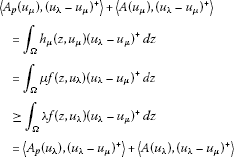(see (3.6) and use the facts that $\mu >\lambda$ and $f⩾0$), so

${\int }_{\left\{{u}_{\lambda }>{u}_{\mu }\right\}}{\left({\parallel \mathrm{\nabla }{u}_{\lambda }\parallel }^{p-2}\mathrm{\nabla }{u}_{\lambda }-{\parallel \mathrm{\nabla }{u}_{\mu }\parallel }^{p-2}\mathrm{\nabla }{u}_{\mu },\mathrm{\nabla }{u}_{\lambda }-\mathrm{\nabla }{u}_{\mu }\right)}_{{\mathbb{R}}^{N}}\phantom{\rule{0.2em}{0ex}}dz+{\parallel \mathrm{\nabla }{\left({u}_{\lambda }-{u}_{\mu }\right)}^{+}\parallel }_{2}^{2}⩽0,$

thus

${|\left\{{u}_{\lambda }>{u}_{\mu }\right\}|}_{N}=0$

and hence ${u}_{\lambda }⩽{u}_{\mu }$.

Therefore, (3.7) becomes

${A}_{p}\left({u}_{\mu }\right)+A\left({u}_{\mu }\right)=\mu {N}_{f}\left({u}_{\mu }\right),$

so

${u}_{\mu }\in S\left(\mu \right)\subseteq int{C}_{+},$

hence $\mu \in \mathcal{Y}$. This proves that $\left[\lambda ,+\mathrm{\infty }\right)\subseteq \mathcal{Y}$. □

Proposition 3.6 If hypotheses H hold, then for every $\lambda >{\lambda }_{\ast }$ problem ${\left(P\right)}_{\lambda }$ has at least two positive solutions

${u}_{0},\stackrel{ˆ}{u}\in int{C}_{+},\phantom{\rule{1em}{0ex}}{u}_{0}\ne \stackrel{ˆ}{u}.$

Proof Note that Proposition 3.5 implies that $\left({\lambda }_{\ast },+\mathrm{\infty }\right)\subseteq \mathcal{Y}$. Let ${\lambda }_{\ast }<\vartheta <\lambda <\mu$. Then we can find ${u}_{\vartheta }\in S\left(\vartheta \right)\subseteq int{C}_{+}$ and ${u}_{\mu }\in S\left(\mu \right)\subseteq int{C}_{+}$. We have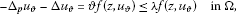(3.8)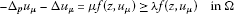(3.9)

(recall that $f⩾0$ and $\vartheta <\lambda <\mu$). As in the proof of Proposition 3.5, we can show that ${u}_{\vartheta }⩽{u}_{\mu }$. We introduce the following truncation of the reaction in problem ${\left(P\right)}_{\lambda }$:

(3.10)

This is a Carathéodory function. We set

${G}_{\lambda }\left(z,\zeta \right)={\int }_{0}^{\zeta }{g}_{\lambda }\left(z,s\right)\phantom{\rule{0.2em}{0ex}}ds$

and consider the ${C}^{1}$-functional ${\stackrel{ˆ}{\psi }}_{\lambda }:{W}_{0}^{1,p}\left(\mathrm{\Omega }\right)⟶\mathbb{R}$ defined by

${\stackrel{ˆ}{\psi }}_{\lambda }\left(u\right)=\frac{1}{p}{\parallel \mathrm{\nabla }u\parallel }_{p}^{p}+\frac{1}{2}{\parallel \mathrm{\nabla }u\parallel }_{2}^{2}-{\int }_{\mathrm{\Omega }}{G}_{\lambda }\left(z,u\right)\phantom{\rule{0.2em}{0ex}}dz\phantom{\rule{1em}{0ex}}\mathrm{\forall }u\in {W}_{0}^{1,p}\left(\mathrm{\Omega }\right).$

It is clear from (3.10) that ${\stackrel{ˆ}{\psi }}_{\lambda }$ is coercive. Also, it is sequentially weakly lower semicontinuous. So, we can find ${u}_{0}\in {W}_{0}^{1,p}\left(\mathrm{\Omega }\right)$ such that

${\stackrel{ˆ}{\psi }}_{\lambda }\left({u}_{0}\right)=\underset{u\in {W}_{0}^{1,p}\left(\mathrm{\Omega }\right)}{inf}{\stackrel{ˆ}{\psi }}_{\lambda }\left(u\right),$

so

${\stackrel{ˆ}{\psi }}_{\lambda }^{\prime }\left({u}_{0}\right)=0$

and thus

${A}_{p}\left({u}_{0}\right)+A\left({u}_{0}\right)={N}_{{g}_{\lambda }}\left({u}_{0}\right).$
(3.11)

Acting on (3.11) with ${\left({u}_{\vartheta }-{u}_{0}\right)}^{+}\in {W}_{0}^{1,p}\left(\mathrm{\Omega }\right)$ and next with ${\left({u}_{0}-{u}_{\mu }\right)}^{+}\in {W}_{0}^{1,p}\left(\mathrm{\Omega }\right)$ (similarly as in the proof of Proposition 3.5), we get

${u}_{\vartheta }⩽{u}_{0}⩽{u}_{\mu }.$

Hence, we have

${u}_{0}\in \phantom{\rule{0.25em}{0ex}}\left[{u}_{\vartheta },{u}_{\mu }\right],$

where .

Then (3.11) becomes

${A}_{p}\left({u}_{0}\right)+A\left({u}_{0}\right)=\lambda {N}_{f}\left({u}_{0}\right)$

(see (3.10)), so

${u}_{0}\in S\left(\lambda \right)\subseteq int{C}_{+}.$

Let

$a\left(y\right)={\parallel y\parallel }^{p-2}y+y\phantom{\rule{1em}{0ex}}\mathrm{\forall }y\in {\mathbb{R}}^{N}.$

Then $a\in {C}^{1}\left({\mathbb{R}}^{N};{\mathbb{R}}^{N}\right)$ (recall that $p>2$) and

$\mathrm{\nabla }a\left(y\right)={\parallel y\parallel }^{p-2}\left(I+\left(p-2\right)\frac{y\otimes y}{{\parallel y\parallel }^{2}}\right)+I\phantom{\rule{1em}{0ex}}\mathrm{\forall }y\in {\mathbb{R}}^{N},$

so

${\left(\mathrm{\nabla }a\left(y\right)\xi ,\xi \right)}_{{\mathbb{R}}^{N}}⩾{\parallel \xi \parallel }^{2}\phantom{\rule{1em}{0ex}}\mathrm{\forall }y,\xi \in {\mathbb{R}}^{N}.$

Note that

$diva\left(\mathrm{\nabla }u\right)={\mathrm{\Delta }}_{p}u+\mathrm{\Delta }u\phantom{\rule{1em}{0ex}}\mathrm{\forall }u\in {W}_{0}^{1,p}\left(\mathrm{\Omega }\right).$

So, we can apply the tangency principle of Pucci and Serrin [, p.35] and infer that

${u}_{\vartheta }\left(z\right)<{u}_{0}\left(z\right)\phantom{\rule{1em}{0ex}}\mathrm{\forall }z\in \mathrm{\Omega }.$
(3.12)

Let $\varrho ={\parallel {u}_{0}\parallel }_{\mathrm{\infty }}$ and let ${\xi }_{\varrho }>0$ be as postulated by hypothesis H(iv). Then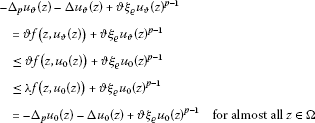(see hypothesis H(iv) and use the facts that $\lambda >\vartheta$ and $f⩾0$), so

${u}_{0}-{u}_{\vartheta }\in int{C}_{+}$
(3.13)

(see (3.12) and Proposition 2.3).

In a similar fashion, we show that

${u}_{\mu }-{u}_{0}\in int{C}_{+}.$
(3.14)

From (3.13) and (3.14), it follows that

${u}_{0}\in {int}_{{C}_{0}^{1}\left(\overline{\mathrm{\Omega }}\right)}\left[{u}_{\vartheta },{u}_{\mu }\right].$
(3.15)

From (3.10), we see that

${\phi }_{\lambda }{|}_{\left[{u}_{\vartheta },{u}_{\mu }\right]}={\stackrel{ˆ}{\psi }}_{\lambda }{|}_{\left[{u}_{\vartheta },{u}_{\mu }\right]}+{\xi }_{\lambda }^{\ast }$

for some ${\xi }_{\lambda }^{\ast }\in \mathbb{R}$.

So, (3.15) implies that ${u}_{0}$ is a local ${C}_{0}^{1}\left(\overline{\mathrm{\Omega }}\right)$-minimizer of ${\phi }_{\lambda }$. Invoking Proposition 2.3, we have that

(3.16)

Hypotheses H(i), (ii) and (iii) imply that for given $\epsilon >0$ and $r>p$, we can find ${c}_{2}={c}_{2}\left(\epsilon ,r\right)>0$ such that

(3.17)

Then for all $u\in {W}_{0}^{1,p}\left(\mathrm{\Omega }\right)$, we have

$\begin{array}{rcl}{\phi }_{\lambda }\left(u\right)& =& \frac{1}{p}{\parallel \mathrm{\nabla }u\parallel }_{p}^{p}+\frac{1}{2}{\parallel \mathrm{\nabla }u\parallel }_{2}^{2}-\lambda {\int }_{\mathrm{\Omega }}F\left(z,u\right)\phantom{\rule{0.2em}{0ex}}dz\\ ⩾& \frac{1}{p}{\parallel \mathrm{\nabla }u\parallel }_{p}^{p}+\frac{1}{2}{\parallel \mathrm{\nabla }u\parallel }_{2}^{2}-\frac{\lambda \epsilon }{p}{\parallel {u}^{+}\parallel }_{p}^{p}-\lambda {c}_{2}{\parallel {u}^{+}\parallel }_{r}^{r}\\ ⩾& \frac{1}{p}\left(1-\frac{\lambda \epsilon }{{\stackrel{ˆ}{\lambda }}_{1}\left(p\right)}\right){\parallel \mathrm{\nabla }u\parallel }_{p}^{p}+\frac{1}{2}{\parallel \mathrm{\nabla }u\parallel }_{2}^{2}-\lambda {c}_{3}{\parallel u\parallel }^{r}\\ ⩾& \frac{1}{p}\left(1-\frac{\lambda \epsilon }{{\stackrel{ˆ}{\lambda }}_{1}\left(p\right)}\right){\parallel \mathrm{\nabla }u\parallel }^{p}-\lambda {c}_{3}{\parallel u\parallel }^{r}\end{array}$
(3.18)

for some ${c}_{3}>0$ (see (3.17) and (2.3)).

Choose $\epsilon \in \left(0,\frac{{\stackrel{ˆ}{\lambda }}_{1}\left(p\right)}{\lambda }\right)$. Then, from (3.18) and since $r>p$, we infer that ${u}_{0}$ is a local minimizer of ${\phi }_{\lambda }$. Without any loss of generality, we may assume that ${\phi }_{\lambda }\left(0\right)=0⩽{\phi }_{\lambda }\left({u}_{0}\right)$ (the analysis is similar if the opposite inequality holds). By virtue of (3.16), as in Gasinski and Papageorgiou  (see the proof of Theorem 2.12), we can find $0<\varrho <\parallel {u}_{0}\parallel$ such that

${\phi }_{\lambda }\left(0\right)=0⩽{\phi }_{\lambda }\left({u}_{0}\right)
(3.19)

Recall that ${\phi }_{\lambda }$ is coercive, hence it satisfies the Palais-Smale condition. This fact and (3.19) permit the use of the mountain pass theorem (see Theorem 2.1). So, we can find $\stackrel{ˆ}{u}\in {W}_{0}^{1,p}\left(\mathrm{\Omega }\right)$ such that

${\eta }_{\vartheta }^{\lambda }⩽{\phi }_{\lambda }\left(\stackrel{ˆ}{u}\right)$
(3.20)

and

${\phi }_{\lambda }^{\prime }\left(\stackrel{ˆ}{u}\right)=0.$
(3.21)

From (3.20) and (3.19), we have that $\stackrel{ˆ}{u}\ne 0$, $\stackrel{ˆ}{u}={u}_{0}$. From (3.21), it follows that $\stackrel{ˆ}{u}\in S\left(\lambda \right)\subseteq int{C}_{+}$. □

Next, we examine what happens at the critical parameter ${\lambda }_{\ast }$.

Proposition 3.7 If hypotheses H hold, then ${\lambda }_{\ast }\in \mathcal{Y}$.

Proof Let ${\left\{{\lambda }_{n}\right\}}_{n⩾1}\subseteq \mathcal{Y}$ be a sequence such that

${\lambda }_{\ast }<{\lambda }_{n}\phantom{\rule{1em}{0ex}}\mathrm{\forall }n⩾1$

and

For every $n⩾1$, we can find ${u}_{n}\in int{C}_{+}$, such that

${A}_{p}\left({u}_{n}\right)+A\left({u}_{n}\right)={\lambda }_{n}{N}_{f}\left({u}_{n}\right).$
(3.22)

We claim that the sequence ${\left\{{u}_{n}\right\}}_{n⩾1}\subseteq {W}_{0}^{1,p}\left(\mathrm{\Omega }\right)$ is bounded. Arguing indirectly, suppose that the sequence ${\left\{{u}_{n}\right\}}_{n⩾1}\subseteq {W}_{0}^{1,p}\left(\mathrm{\Omega }\right)$ is unbounded. By passing to a suitable subsequence if necessary, we may assume that $\parallel {u}_{n}\parallel ⟶+\mathrm{\infty }$. Let

${y}_{n}=\frac{{u}_{n}}{\parallel {u}_{n}\parallel }\phantom{\rule{1em}{0ex}}\mathrm{\forall }n⩾1.$

Then $\parallel {y}_{n}\parallel =1$ and ${y}_{n}\in int{C}_{+}$ for all $n⩾1$. From (3.22), we have

${A}_{p}\left({y}_{n}\right)+\frac{1}{{\parallel {u}_{n}\parallel }^{p-2}}A\left({y}_{n}\right)=\frac{{\lambda }_{n}{N}_{f}\left({u}_{n}\right)}{{\parallel {u}_{n}\parallel }^{p-1}}\phantom{\rule{1em}{0ex}}\mathrm{\forall }n⩾1.$
(3.23)

Recall that

(see (3.1)), so the sequence ${\left\{\frac{{N}_{f}\left({u}_{n}\right)}{{\parallel {u}_{n}\parallel }^{p-1}}\right\}}_{n⩾1}\subseteq {L}^{{p}^{\prime }}\left(\mathrm{\Omega }\right)$ is bounded. This fact and hypothesis H(ii) imply that at least for a subsequence, we have

(3.24)

(see Gasinski and Papageorgiou ). Also, passing to a subsequence if necessary, we may assume that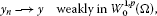(3.25)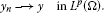(3.26)

On (3.23) we act with ${y}_{n}-y\in {W}_{0}^{1,p}\left(\mathrm{\Omega }\right)$, pass to the limit as $n\to +\mathrm{\infty }$ and use (3.24) and (3.26). Then

$\underset{n\to +\mathrm{\infty }}{lim}\left(〈{A}_{p}\left({y}_{n}\right),{y}_{n}-y〉+\frac{1}{{\parallel {u}_{n}\parallel }^{p-2}}〈A\left({y}_{n}\right),{y}_{n}-y〉\right)=0,$

so

$\underset{n\to +\mathrm{\infty }}{lim}〈{A}_{p}\left({y}_{n}\right),{y}_{n}-y〉⩽0.$

Using Proposition 2.4, we have that

and so

$\parallel y\parallel =1.$
(3.27)

Passing to the limit as $n\to +\mathrm{\infty }$ in (3.23) and using (3.24), (3.27) and the fact that $p>2$, we obtain

${A}_{p}\left(y\right)=0,$

so $y=0$, which contradicts (3.27).

This proves that the sequence ${\left\{{u}_{n}\right\}}_{n⩾1}\subseteq {W}_{0}^{1,p}\left(\mathrm{\Omega }\right)$ is bounded. So, passing to a subsequence if necessary, we may assume that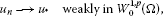(3.28)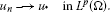(3.29)

On (3.22) we act with ${u}_{n}-{u}_{\ast }\in {W}_{0}^{1,p}\left(\mathrm{\Omega }\right)$, pass to the limit as $n\to +\mathrm{\infty }$ and use (3.28) and (3.29). Then

$\underset{n\to +\mathrm{\infty }}{lim}\left(〈{A}_{p}\left({u}_{n}\right),{u}_{n}-{u}_{\ast }〉+〈A\left({u}_{n}\right),{u}_{n}-{u}_{\ast }〉\right)=0,$

so

$\underset{n\to +\mathrm{\infty }}{lim sup}〈{A}_{p}\left({u}_{n}\right),{u}_{n}-{u}_{\ast }〉⩽0$

(since A is monotone) and thus

(3.30)

(see Proposition 2.4).

Therefore, if in (3.22) we pass to the limit as $n\to +\mathrm{\infty }$ and use (3.30), then

${A}_{p}\left({u}_{\ast }\right)+A\left({u}_{\ast }\right)={\lambda }_{\ast }{N}_{f}\left({u}_{\ast }\right)$

and so ${u}_{\ast }\in {C}_{+}$ is a solution of problem ${\left(P\right)}_{{\lambda }_{\ast }}$.

We need to show that ${u}_{\ast }\ne 0$. From (3.22), we have

From Ladyzhenskaya and Uraltseva [, p.286], we know that we can find ${M}_{1}>0$ such that

${\parallel {u}_{n}\parallel }_{\mathrm{\infty }}⩽{M}_{1}\phantom{\rule{1em}{0ex}}\mathrm{\forall }n⩾1.$

Then applying Theorem 1 of Lieberman , we can find $\beta \in \left(0,1\right)$ and ${M}_{2}>0$ such that

${u}_{n}\in {C}_{0}^{1,\beta }\left(\overline{\mathrm{\Omega }}\right)\phantom{\rule{1em}{0ex}}\text{and}\phantom{\rule{1em}{0ex}}{\parallel {u}_{n}\parallel }_{{C}_{0}^{1,\beta }\left(\overline{\mathrm{\Omega }}\right)}⩽{M}_{2}\phantom{\rule{1em}{0ex}}\mathrm{\forall }n⩾1.$

Recall that ${C}_{0}^{1,\beta }\left(\overline{\mathrm{\Omega }}\right)$ is embedded compactly in ${C}_{0}^{1}\left(\overline{\mathrm{\Omega }}\right)$. So, by virtue of (3.28), we have

Suppose that ${u}_{\ast }=0$. Then

(3.31)

Hypothesis H(iii) implies that for a given $\epsilon >0$, we can find $\delta \in \left(0,\epsilon \right]$ such that

(3.32)

From (3.31), it follows that we can find ${n}_{0}⩾1$ such that

(3.33)

Therefore, for almost all $z\in \mathrm{\Omega }$ and all $n⩾{n}_{0}$, we have

$-{\mathrm{\Delta }}_{p}{u}_{n}\left(z\right)-\mathrm{\Delta }{u}_{n}\left(z\right)={\lambda }_{n}f\left(z,{u}_{n}\left(z\right)\right)⩽{\lambda }_{n}\epsilon {u}_{n}{\left(z\right)}^{p-1}$

(see (3.32) and (3.33)), so

${\parallel \mathrm{\nabla }{u}_{n}\parallel }_{p}^{p}⩽{\lambda }_{n}\epsilon {\parallel {u}_{n}\parallel }_{p}^{p}⩽\frac{{\lambda }_{n}}{{\stackrel{ˆ}{\lambda }}_{1}\left(p\right)}\epsilon {\parallel \mathrm{\nabla }{u}_{n}\parallel }_{p}^{p}\phantom{\rule{1em}{0ex}}\mathrm{\forall }n⩾{n}_{0}$

(see (2.3)), thus

$\frac{{\stackrel{ˆ}{\lambda }}_{1}\left(p\right)}{\epsilon }⩽{\lambda }_{n}\phantom{\rule{1em}{0ex}}\mathrm{\forall }n⩾{n}_{0}$

and so

$\frac{{\stackrel{ˆ}{\lambda }}_{1}\left(p\right)}{\epsilon }⩽{\lambda }_{\ast }.$

Let $\epsilon ↘0$ to get a contradiction. This proves that ${u}_{\ast }\ne 0$ and so ${u}_{\ast }\in S\left({\lambda }_{\ast }\right)\subseteq int{C}_{+}$, hence ${\lambda }_{\ast }\in \mathcal{Y}$. □

The bifurcation-type theorem summarizes the situation for problem ${\left(P\right)}_{\lambda }$.

Theorem 3.8 If hypotheses H hold, then there exists ${\lambda }_{\ast }>0$ such that

(a) for every $\lambda >{\lambda }_{\ast }$ problem ${\left(P\right)}_{\lambda }$ has at least two positive solutions:

${u}_{0},\stackrel{ˆ}{u}\in int{C}_{+};$

(b) for $\lambda ={\lambda }_{\ast }$ problem ${\left(P\right)}_{\lambda }$ has at least one positive solution ${u}_{\ast }\in int{C}_{+}$;

(c) for $\lambda \in \left(0,{\lambda }_{\ast }\right)$ problem ${\left(P\right)}_{\lambda }$ has no positive solution.

Remark 3.9 As the referee pointed out, it is an interesting problem to produce an example in which, at the bifurcation point ${\lambda }^{\ast }>0$, the equation has exactly one solution. We believe that the recent paper of Gasiński and Papageorgiou  on the existence and uniqueness of positive solutions will be helpful. Concerning the existence of nodal solutions for $\lambda \in \left(0,{\lambda }^{\ast }\right)$, we mention the recent paper of Gasiński and Papageorgiou , which studies the $\left(p,2\right)$-equations and produces nodal solutions for them.

## References

1. Maya C, Shivaji R: Multiple positive solutions for a class of semilinear elliptic boundary value problems. Nonlinear Anal. 1999, 38: 497-504. 10.1016/S0362-546X(98)00211-9

2. Rabinowitz PH: Pairs of positive solutions of nonlinear elliptic partial differential equations. Indiana Univ. Math. J. 1973, 23: 173-186. 10.1512/iumj.1973.23.23014

3. Guo Z: Some existence and multiplicity results for a class of quasilinear elliptic eigenvalue problems. Nonlinear Anal. 1992, 18: 957-971. 10.1016/0362-546X(92)90132-X

4. Hu S, Papageorgiou NS: Multiple positive solutions for nonlinear eigenvalue problems with the p -Laplacian. Nonlinear Anal. 2008, 69: 4286-4300. 10.1016/j.na.2007.10.053

5. Perera K: Multiple positive solutions of a class of quasilinear elliptic boundary value problems. Electron. J. Differ. Equ. 2003, 7: 1-5.

6. Benci V, D’Avenia P, Fortunato D, Pisani L: Solitons in several space dimensions: Derrick’s problem and infinitely many solutions. Arch. Ration. Mech. Anal. 2000, 154: 297-324. 10.1007/s002050000101

7. Cingolani S, Degiovanni M: Nontrivial solutions for p -Laplace equations with right hand side having p -linear growth at infinity. Commun. Partial Differ. Equ. 2005, 30: 1191-1203. 10.1080/03605300500257594

8. Sun M: Multiplicity of solutions for a class of the quasilinear elliptic equations at resonance. J. Math. Anal. Appl. 2012, 386: 661-668. 10.1016/j.jmaa.2011.08.030

9. Marano SA, Papageorgiou NS: Multiple solutions to a Dirichlet problem with p -Laplacian and nonlinearity depending on a parameter. Adv. Nonlinear Stud. 2012, 3: 257-275.

10. Marano SA, Papageorgiou NS:Constant-sign and nodal solutions of coercive $\left(p,q\right)$-Laplacian problems. Nonlinear Anal. 2013, 77: 118-129.

11. Gasiński L, Papageorgiou NS: Nonlinear Analysis. Chapman & Hall/CRC, Boca Raton; 2006.

12. Mawhin J, Willem M: Origin and evolution of the Palais-Smale condition in critical point theory. J. Fixed Point Theory Appl. 2010, 7: 265-290. 10.1007/s11784-010-0019-7

13. Gasiński L, Papageorgiou NS: Multiple solutions for nonlinear coercive problems with a nonhomogeneous differential operator and a nonsmooth potential. Set-Valued Var. Anal. 2012, 20: 417-443. 10.1007/s11228-011-0198-4

14. Brézis H, Nirenberg L:${H}^{1}$ versus ${C}^{1}$ local minimizers. C. R. Acad. Sci., Sér. 1 Math. 1993, 317: 465-472.

15. Arcoya D, Ruiz D: The Ambrosetti-Prodi problem for the p -Laplace operator. Commun. Partial Differ. Equ. 2006, 31: 849-865. 10.1080/03605300500394447

16. Dinca G, Jebelean P, Mawhin J: Variational and topological methods for Dirichlet problems with p -Laplacian. Port. Math. 2001, 58: 339-378.

17. Ladyzhenskaya OA, Uraltseva N Mathematics in Science and Engineering 46. In Linear and Quasilinear Elliptic Equations. Academic Press, New York; 1968.

18. Lieberman GM: Boundary regularity for solutions of degenerate elliptic equations. Nonlinear Anal. 1988, 12: 1203-1219. 10.1016/0362-546X(88)90053-3

19. Pucci P, Serrin J: The Maximum Principle. Birkhäuser, Basel; 2007.

20. Gasiński L, Papageorgiou NS: Nodal and multiple constant sign solutions for resonant p -Laplacian equations with a nonsmooth potential. Nonlinear Anal. 2009, 71: 5747-5772. 10.1016/j.na.2009.04.063

21. Gasiński L, Papageorgiou NS: Existence and uniqueness of positive solutions for the Neumann p -Laplacian. Positivity 2012. doi:10.1007/s11117-012-0168-6 (published online)

22. Gasiński, L, Papageorgiou, NS: Multiplicity theorems for $\left(p,2\right)$-equations (submitted)

## Acknowledgements

Dedicated to Professor Jean Mawhin on the occasion of his 70th birthday.

The authors would like to express their gratitude to both knowledgeable referees for their corrections and remarks. This research has been partially supported by the Ministry of Science and Higher Education of Poland under Grants no. N201 542438 and N201 604640.

## Author information

Authors

### Corresponding author

Correspondence to Leszek Gasiński.

### Competing interests

The authors declare that they have no competing interests.

### Authors’ contributions

The authors declare that the work was realized in collaboration with the same responsibility. All authors read and approved the final manuscript.

## Rights and permissions

Reprints and Permissions

Gasiński, L., Papageorgiou, N.S. Multiplicity of positive solutions for eigenvalue problems of $\left(p,2\right)$-equations. Bound Value Probl 2012, 152 (2012). https://doi.org/10.1186/1687-2770-2012-152

• Accepted:

• Published:

• DOI: https://doi.org/10.1186/1687-2770-2012-152

### Keywords

• nonlinear regularity
• tangency principle
• p-Laplacian
• bifurcation-type theorem
• positive solutions